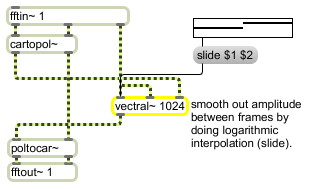# vectral~

Vector-based envelope follower

## Description

vectral~ is useful for filtering frame based signal data such as the output of fft~. It may operate in one of the following modes:
rampsmooth <up> <down>- linear ramp across up/down frames to the new value
slide <up> <down>- logarithmic movement to new value
deltaclip <max> <min>- limits the change in samples to be in the given range

## Arguments

Name Type Opt Description
vector-size int opt The argument is the vector size for the operation. It defaults to 512, but should be set appropriately for the size of the vectors you feed into the vectral~ object.

## Messages

 clear Clears object's sample memory (in case of a blow-up). deltaclip limits-of-change (2 floats) [list] In left inlet: The word deltaclip, followed by two floats, limits the change in bins of successive vectors to the values given. This is equivalent to the time-domain deltaclip~ object. rampsmooth number-of-interpolating-frames [list] In left inlet: The word rampsmooth, followed by two ints, causes the vector to be smoothed in a linear fashion across successive frames. The arguments specify the number of frames to use to interpolate values in both directions. This is equivalent to the time-domain filtering done by the rampsmooth~ object. signal In left inlet: Accepts a sync signal for the output index of the vector. This is typically in the range of 0 to n-1 where n is the size of the vector. In middle inlet: A sync signal received in the middle inlet is used to synchronize the input index of the vector being processed. The sync signal will typically be in the range 0 to n-1 where n is the size of the vector. If the range of the sync signal is different than the output index, the incoming vector will be "bin-shifted" by the difference between the two signals. In right inlet: Signal data to be filtered. This will usually be frequency-domain information such as the output of an fft~ or fftin~ object. size vector-size [int] In left inlet: The word size, followed by a number, sets the vector size for the operation. The default is 512. slide slide-denominator-coefficients (2 floats) [list] In left inlet: The word slide, followed by two floats, causes vectral~ to do logarithmic interpolation of successive vectors in a manner equivalent to the time-domain slide~ object. The two arguments determine the denominator coefficient for the amount of the slide.

## Output

signal: A smoothed version of the signal input into the right inlet, according to the parameters given to the vectral~ object.

## Examplesvectral~ performs different types of smoothing between frames of vectored data (e.g. FFT signals)

Name Description
cartopol Cartesian to Polar coordinate conversion
cartopol~ Signal Cartesian to Polar coordinate conversion
deltaclip~ Limit changes in signal amplitude
fft~ Fast Fourier transform
fftin~ Input for a patcher loaded by pfft~